8.7

## Lecture 9: Global Function Definitions and Non-tail Calls

We’ve seen how to call into Rust code using the System V AMD64 calling convention, and we’ve seen how code using only tail calls can be compiled directly to efficient assembly using only jumps. Today we will extend our source language to include non-tail calls, and we will introduce our own internal calling convention

### 1Non-Tail Calls and the Stack

Tail calls were great because they could be compiled efficiently as a jump, but most calls in a program aren’t that simple. In a tail call, we call another function and produce whatever value it produced, whereas in a non-tail call, we use some other function as a sub-procedure to produce some intermediate value that we then use for some other purpose. For instance consider the following program which for simplicity we’ve already written in sequential form:

def max(x,y): let b = x >= y in if b: x else: y in let z = max(10, 4) in let q = max(3, 8) in z * q

Here we use max as a convenient sub-procedure to compute the maximum of two numbers that we then use multiple times with different inputs in the main expression of our program. If we think about how we will compile these expressions something stands out. We store local variables and arguments on the stack, so z and q will be placed at [RSP - 8 * 1], [RSP - 8 * 2] respectively, but also in the body of max we place x and y using the same offsets: [RSP - 8 * 1], [RSP - 8 * 2]. So if whenever we make a call we jmp into the code for max, won’t our local variables be overwritten?

Do Now!

How can we ensure that the variables x,y don’t overwrite the values of z,q?

Well notice that while the literal code for the address is the same, the address [RSP - 8 * n] is relative to the value of RSP. This is the difference betweeen a local variable and a global variable, which would have a fixed globally known address in memory. So to implement a function call, we need to teach our functions how to share the memory allocated to the process. If we recall what the stack looks like: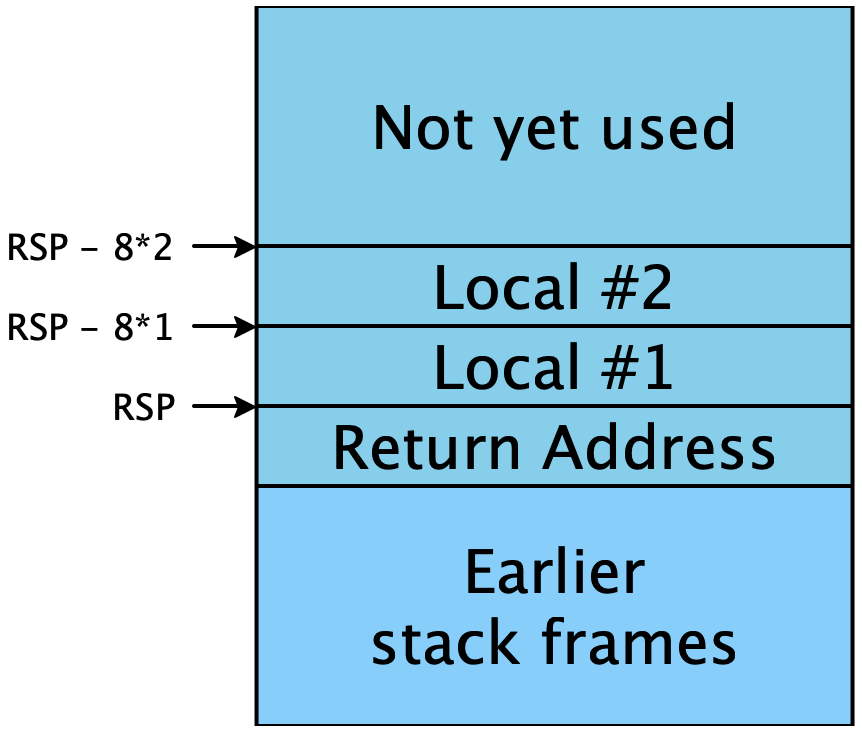The stack pointer RSP points to the base of our current stack frame, which is our current return address. Below that (higher addresses) we have our caller’s stack frames, which we are careful not to overwrite. In our stack frame we store our local variables, but above that we have free space. When we make a non-tail call, we will ensure that the base of the callee’s stack frame is above all of our local variables, and so if the callee follows our stack protocol, it will be sure not to overwrite any of our stack frame. Additionally, we need to provide a new return address, which will be stored at the base of the callee’s stack frame: an address back to our code so that when the callee returns the code after the call will execute.

So for example we can compile the above code as follows:

max:
;; as previously
start_here:
mov [RSP - 8 * 2], 20 ;; store the first argument (10)
mov [RSP - 8 * 3], 8  ;; store the second argument (4)
mov [RSP - 8 * 1], RAX ;; store the result in z
mov [RSP - 8 * 3], 6   ;; store the first argument (3)
mov [RSP - 8 * 4], 16  ;; store the second argument (8)
sub RSP, 8             ;; move RSP so as not to overwrite our local variable z
call max
mov [RSP - 8 * 2], RAX ;; store the result in q
mov RAX, [RSP - 8 * 1] ;; load z
add RAX, [RSP - 8 * 2] ;; multiply z * q
ret                    ;; return the result

At the beginning of start_here, the RSP is pointing to the return address from our Rust main function. We then mov the two arguments onto the stack and call max, when we start executing max the stack looks like

Let’s step through what the stack looks like at each point.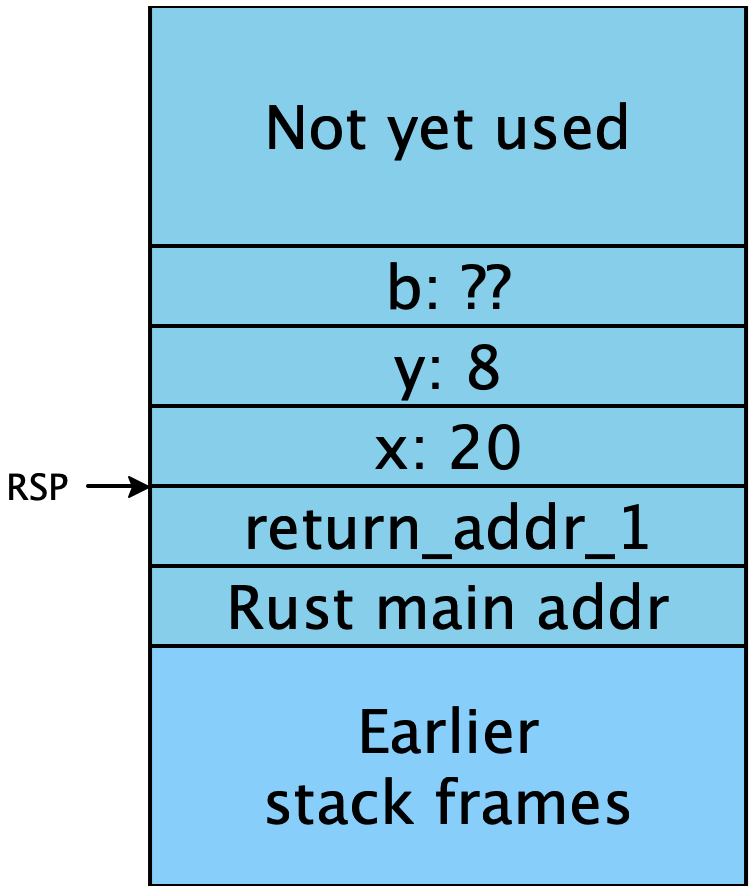That is, the stack pointer is pointing to the new return address, return_addr_1 that we just pushed, and the implementation of max can freely use the space above it. Then when max returns, we will resume at return_addr_1. Note that we don’t actually need to include the labels return_addr_1 and return_addr_2 in the assembly program, the call instruction will push the next address of the next instruction onto the stack even if it doesn’t have a label. We are just including them to be explicit.

The second time we make a call to max, we need to make sure that when we call we don’t overwrite our new local variable z, so before we subtract from RSP so that when we call, the base of the callee’s stack frame is entirely above the caller’s stack frame. Then upon entry into max the second time, the stack looks like this: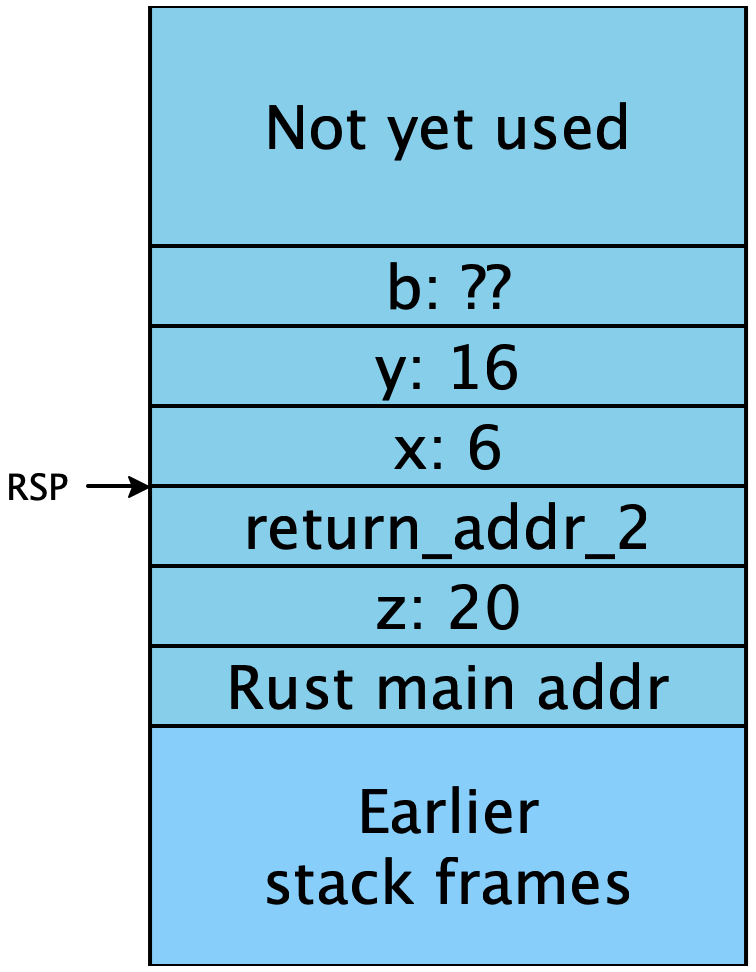So this leads us to the general case for how to compile a non-tail call. Say we have M local variables and we are calling a function f with N arguments x0,x1,.... Then before we start the call the stack looks like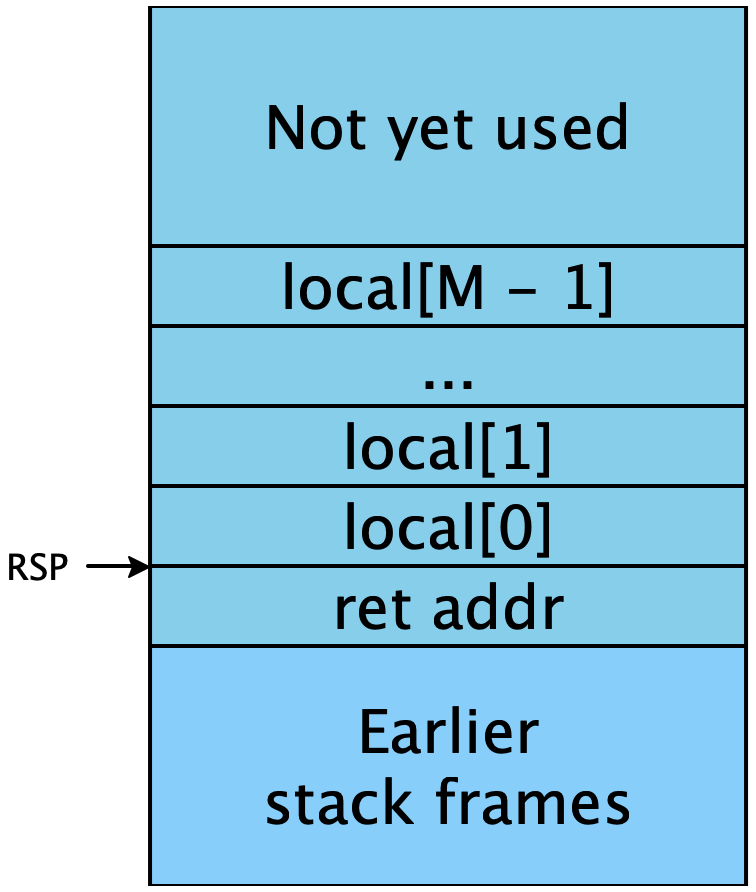Then we should first mov all of the arguments into the space above our stack frame: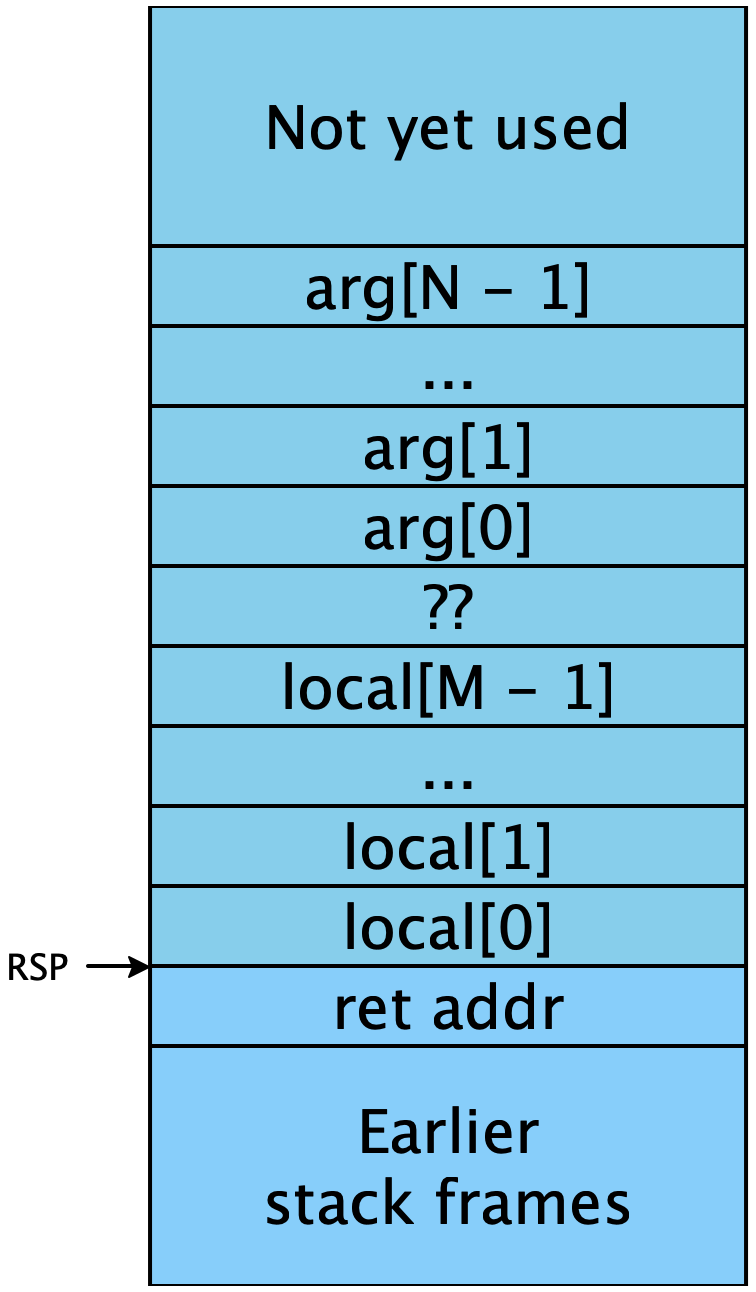Then we should sub from RSP to put it at the right spot so that a call will push the return address to the right place: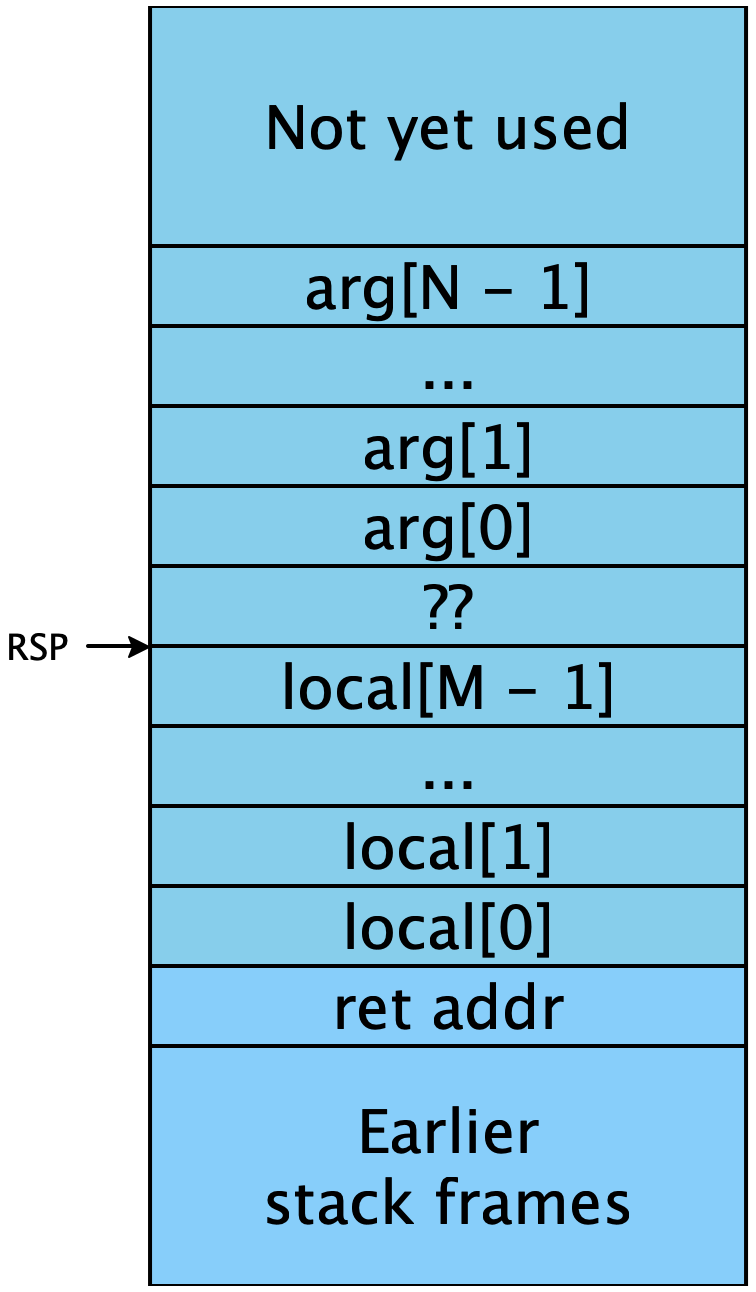And then we execute the call instruction, making sure to add back to RSP so it returns to its original place at the base of our stack frame. In code, this means a call should be compiled as follows:

mov [RSP - 8 * (M + 2 + 0)], arg
mov [RSP - 8 * (M + 2 + 1)], arg
...
mov [RSP - 8 * (M + 2 + N - 1)], arg[N - 1]
sub RSP, 8 * M
call f
add RSP, 8 * M

#### 1.1Snake Calling Convention v0

Let’s codify this approach to compiling function calls into a more formal specification. We’ll call this the Snake Calling Convention v0, as it’s the way we will make internal function calls as opposed to the System V calling convention we use when calling into Rust.

To call a function with N variables:

• Upon entry, RSP should be at an address divisible by 16 and point to the return address.

• The arguments (each 64-bits) will be placed in order in decreasing addresses from RSP with the first argument closest to RSP

• Stack space at higher addresses than RSP belongs to the caller and so should be unchanged when returning.

• To return, place the return value into RAX, decrement RSP by 8 and jump to the caller’s return address (this can be achieved by simply using ret)

• The callee is free to use any other registers as they see fit (i.e., the caller must save the values if they need them).

There are two parts of this calling convention we haven’t covered: first the alignment (which is there to make calling into Rust code easier) and second, the registers. It essentially says we as the callee in the Snake calling convention never have to worry about overwriting registers. Once we start using registers we will need to be more careful about this.

The alignment part is trickier: we say upon entry into a function, we want the address to be equal to 0 modulo 16. Note that this is the exact oppposite of the alignment requirement for System V. While that might sound strange, it should actually make our lives easier: in the common case of a call into Rust that pushes no arguments (and saving no locals), we can just call the code directly. But when we do have to save locals, it means that we should be sure to add extra padding if we would otherwise be misaligned.

Exercise

If we keep RSP at 0 modulo 16 in our Snake code, and Rust code must have RSP equal to 8 modulo 16 upon entry to a function, then when we call from Snake to Rust should we round up our number of locals saved to the nearest even number or odd number?

Also remember that while our internal function definitions are called only using the Snake calling convention, the start_here function is called using the System V calling convention, which is as mentioned above has exactly the wrong alignment. A simple way to do this is to add a "wrapper" around our main expression:

start_here:
call main
ret
main:
;; actual main expression

This way we can compile our main expression as if it were a function with 0 variables and use the same compilation strategy. This also has a negliegible effect on the runtime/space usage of our program.

### 2Local Function Definitions and Lambda Lifting

The above strategy works fine for top-level function definitions, but consider what happens if we have a local function definition:

def multiply(x, y):
def mult_by_x(i):
if i == 0:
0
else:
x + mult_by_x(i - 1)
in
loop(y)
in
multiply(5, 3)

This function multiplies two numbers x and y by repeated addition. It works by defining a recursive function mult_by_x that works by multiplying the fixed variable x to the argument i. Since the recursive call is not a tail call, the stack grows each time a recursive call is made, and so we cannot tell where x will be stored on the stack statically! We say that mult_by_x captures the variable x in its body. To implement this, we want to copy x into mult_by_x’s stack frame on each call. The easiest way to do this is by a program transformation, which will transform all local function definitions into global ones:

def multiply(x, y):
mult_by_x(x, y)
and
def mult_by_x(x, i):
if i == 0:
0
else:
x + mult_by_x(x, i - 1)
in
multiply(5, 3)

This process is called lambda lifting because local function definitions are often constructed using lambda-notation. So if we can do this transformation, we will be able to use the above implementation of non-tail calls. But there are a few pitfalls to lambda lifting that we discuss in class.

Exercise

Implement non-tail calls for globally defined functions in diamondback.